### Home > A2C > Chapter 13 > Lesson 13.1.3 > Problem13-54

13-54.
1. Find the infinite sum (if possible.) Homework Help ✎

1.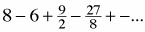2.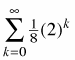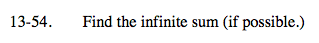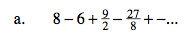Treat as the sum of two different sums.

$t(n)=\frac{128}{9}\left(\frac{9}{16}\right)^n$

$u(n)=-\frac{32}{3}\left(\frac{9}{16}\right)^n$

Use the geometric summation formula on both and add the results.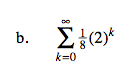Remember the prerequisites of the summation formula.